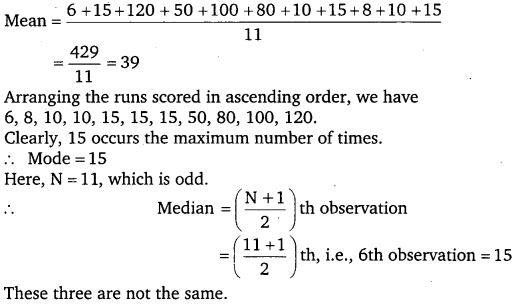# Class 7 Maths NCERT Solutions for Chapter – 3 Data Handling EX – 3.2

## Data Handling

Question 1.
The scores in mathematics test (out of 25) of 15 students is as follows :
19, 25, 23, 20, 9, 20,15, 10, 5,16, 25, 20, 24, 12, 20
Find the mode and median of this data. Are they same?

Solution:
Arranging the scores in Mathematics in ascending order,
we have 5, 9, 10, 12, 15, 16, 19, 20, 20, 20, 20, 23, 24, 25, 25.
Clearly, 20 occurs the maximum number of times.Question 2.
The runs scored in a cricket match by 11 players is as follows :
6, 15, 120, 50, 100, 80, 10, 15, 8, 10,15
Find the mean, mode and median of this data. Are the three same?

Solution:Question 3.
The weights (in kg) of 15 students of a class are :
38, 42, 35, 37, 45, 50, 32, 43, 43, 40, 36, 38, 43, 38, 47
(i) Find the mode and median of this data.
(ii) Is there more than one mode?

Solution:
(i) Arranging the weights (in kg) in ascending order,
we have 32, 35, 36, 37, 38, 38, 38, 40, 42, 43, 43, 43, 45, 47, 50.
Clearly, 38 and 43 occur the maximum number of times.
∴ Modes are 38 and 43.
Here, N = 15, which is odd.(ii) Yes, there are more than one mode.

Question 4.
Find the mode and median of the following data :
13, 16, 12, 14, 19, 12, 14, 13, 14.

Solution:
Arranging the data in ascending order, we have
12, 12, 13, 13, 14, 14, 14, 16, 19
Clearly, 14 occurs the maximum number of times.Question 5.
Tell whether the statement is true or false :

1. The mode is always one of the numbers in a data.
2. The mean is one of the numbers in a data.
3. The median is always One of the numbers in a data.
4. The data 6, 4, 3, 8, 9, 12, 13, 9 has mean 9.

Solution:

1. True
2. False
3. True
4. False